## Text Functions

LEFT, RIGHT,MID, TRIM, LEN, FIND, SEARCH, SUBSTITUTE, REPT etc.,

We will see syntax with examples of each function.

LEFT FUNCTION

The LEFT function returns a string consisting of the specified number of characters from the left end of a given string.

LEFT(text, number)

text :  A string. and is  a string value.

number:  An optional argument specifying the desired length of the returned string. string-length is a number value and must be greater than or equal to 1.

Notes

The count includes all spaces, numbers, and special characters.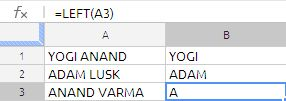the formula in cell B1 is

=LEFT(A1,5)

the formula extracted the first 5 characters of the text string in cell A1 as stated earlier in the notes it has counted the space also.

the formula in cell B3 is

=LEFT(A3)

we have ignored the second argument that means only one character will be extracted

RIGHT FUNCTION

The RIGHT function returns a string consisting of the specified number of characters from the right end of a given string.

RIGHT(text, number)

text:  A string. source-string is a string value.

number:  An optional argument specifying the desired length of the returned string. string-length is a number value and must be greater than or equal to 1.

Notes

If string-length is greater than or equal to the length of source-string, the string

returned is equal to source-string.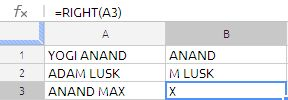the formula in cell B1 is

=RIGHT(A1,6)

the formula extracted the first 6 characters of the text string in cell A1 as stated earlier in the notes it has counted the space also.

the formula in cell B3 is

=RIGHT(A3)

we have ignored the second argument that means only one character will be extracted

MID FUNCTION

The MID function returns a string consisting of the given number of characters from a string starting at the specified position.

MID(text, start, number)

text :  A string. source-string is a string value.

start:  The position within the specified string at which the action should begin. start is a number value that must be greater than or equal to 1 and less than or equal to the number of characters in source-string.

number:  The desired length of the returned string. string-length is a number

value and must be greater than or equal to 1.

Notes

If number is greater than or equal to the length of text, the string returned is equal to text, beginning at start.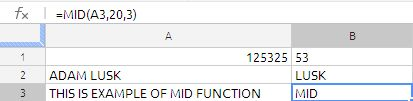The formula in cell B1 is

=MID(A1,3,2)

even though the cell A1 is numeric value still the MID function extract the data but they are in string form that is why the value in the cell B1 is left justified. to convert that string to number we can modify the formula as follows:

=MID(A1,3,2)+0

Similarly the formula in cell B2 is

=MID(A2,6,4)

and formula in cell B3 is

=MID(A3,20,3)

MID function is extensively used along with search, find to extract text.

TRIM FUNCTION

The TRIM function returns a string based on a given string, after removing extra spaces.

TRIM(text)

text:  A string. source-string is a string value.

Notes

TRIM removes all spaces before the first character, all spaces after the last character, and all duplicate spaces between characters, leaving only single spaces between words.

This function is very useful whenever we are importing CSV files into google docs and cleaning the leading and trailing spaces in the string

LEN FUNCTION

The LEN function returns the number of characters in a string. The LEN function very frequently used in google docs spreadsheets when every there is a array formula. it is application is very unique to google spreadsheets.

LEN(text)

text:  A string. source-string is a string value.

Notes

The count includes all spaces, numbers, and special characters.

have look at the following formula structure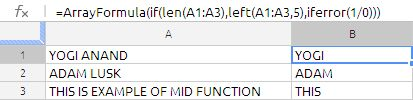in the above formula LEN function is used to restrict the population of the LEFT function to 3 rows.

FIND FUNCTION

The FIND function returns the starting position of one string within another.

FIND(find_text, text, position)

find_text:  The string to find. search-string is a string value.

text:  A string. source-string is a string value.

position:  An optional argument that specifies the position within the specified string at which the action should begin. position is a number value that must be greater than or equal to 1 and less than or equal to the number of characters in find-text.

Notes

The search is case sensitive and spaces are counted. Wildcards are not allowed. To

use wildcards or to ignore case in your search, use the SEARCH function.

SEARCH FUNCTION

The SEARCH function returns the starting position of one string within another, ignoring case and allowing wildcards.

SEARCH(find_text, text, position)

find_text:  The string to find. search-string is a string value.

text :  A string. text is a string value in which we want to search

position:  An optional argument that specifies the position within the specified string at which the action should begin. position is a number value that must be greater than or equal to 1 and less than or equal to the number of characters in source-string.

Notes

Wildcards are permitted in search-string. In search-string, use an * (asterisk) to match multiple characters or a ? (question mark) to match any single character in find_text.

Specifying position permits you to begin the search for text within, rather than at the beginning of, source-string. This is particularly useful if find_text may contain multiple instances of text and you wish to determine the starting

position of other than the first instance. If start-pos is omitted, it is assumed to be 1.

To have case considered in your search, use the FIND function.

Difference Between FIND & SEARCH

1. Both functions find the position of a substring in a string – the position of some characters within a different set of characters.

2. FIND is case sensitive and does not allow wildcards such as * (1 or more characters) or ? a single character.

3. SEARCH is NOT case sensitive and it accepts wildcards.

? Is wildcard for a single character. * Is wildcard for one or more characters.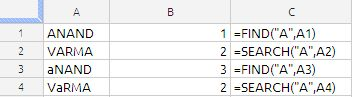in the above image we can clearly see the difference between search and find function in cell B3 & B4,

the Find function failed it identify the “a” in the because it is case sensitive and whereas search could able to identify the “a” position correctly. for all practical purposes we can use the search function instead of find function.

SUBSTITUTE FUNCTION

The SUBSTITUTE function returns a string where the specified characters of a given string have been replaced with a new string.

SUBSTITUTE(text, search_text, new text, occurrence)

text:  A string. source-string is a string value.

search_text:  The string within the given string that is to be replaced. search_text string is a string value.

new text:  The text used as a replacement for the section of the given string that is replaced. new-string is a string value. It does not have to be the same length as the text replaced.

occurrence:  An optional value specifying the occurrence that should be replaced. occurrence is a number value and must be greater than or equal to 1, or omitted. If greater than the number of times existing-string appears within source-string, no replacement will occur. If omitted, all occurrences of existing-string within source string  will be replaced by new-string.

Notes

You can replace individual characters, whole words, or strings of characters within words.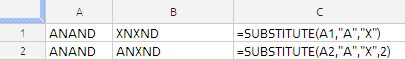in the above example the character “A” is replaced with “X” in cell B1 and in cell B2 we have replaced the second occurrence of “A” has been replaced with “X”.

the substitution function is widely used to extract the certain part of the string along with other function such as Find, Search, MID etc.

Example 1:

Substitute is wide used to count spaces

suppose A1 hold

This  is an example for counting spaces

=LEN(A1)-LEN(SUBSTITUTE(A1,” “,””))

Example 2:

:If we are extracting the text from the 1234567890abcd we have to use the substitute function in other spreadsheet applications the formula will be is as follows:

=substitute(substitute(substitute(substitute(substitute(substitute(substitute(substitute(substitute(SUBSTITUTE(F1,1,””),2,””),3,””),4,””),5,””),6,””),7,””),8,””),9,””),0,””)

fortunately the Google docs spreadsheet supports the regular expression the formula will in cell H1 is

=REGEXREPLACE(F1,”[0-9]”,””)

Example 3: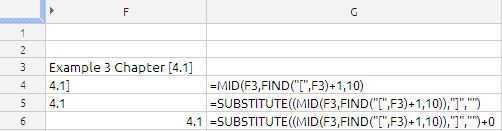Our objective is to extract 4.1 which is in the square brackets.

to extract this we have started with MID function to identify the start point we have used the find function to identify the position and added 1 to avoid the left square bracket

we have removed the right square bracket by using the substitute function by replacing the “]” with a blank

now you can clearly see 4.1 the in Cell F5, but the problem is it is left justified that means it is text.

to convert the text to number we just added 0 (zero) to the formula.

the final formula is

=SUBSTITUTE((MID(F3,FIND(“[“,F3)+1,10)),”]”,””)+0

REPT FUNCTION

The REPT function returns a string that contains a given string repeated a specified number of times.

REPT(text, number)

text:  A string. text is a string value.

number:  The number of times the given string should be repeated. repeat number is a number value that must be greater than or equal to 0.

the REPT function is also used to create a Histogram as shown in the below image.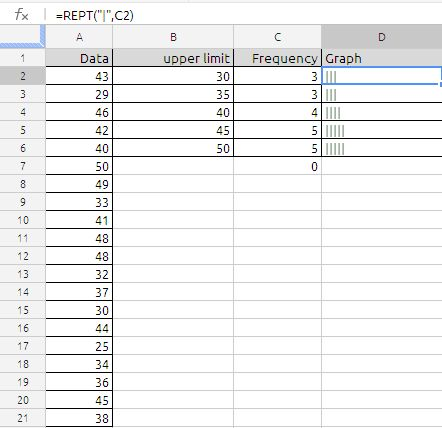Data is in cell A2:A21,

the formula in cell C1 is

=FREQUENCY(A2:A21,B2:B6)

formula in cell D1 is

=REPT(“|”,C2) and drag it down